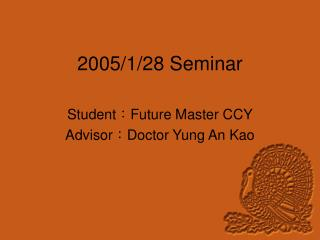DownloadDownload Presentation2005/1/28 Seminar

# 2005/1/28 Seminar

Télécharger la présentation## 2005/1/28 Seminar

- - - - - - - - - - - - - - - - - - - - - - - - - - - E N D - - - - - - - - - - - - - - - - - - - - - - - - - - -
##### Presentation Transcript

1. 2005/1/28 Seminar Student：Future Master CCY Advisor：Doctor Yung An Kao

2. Outlines • Introduction • Case Discussion • Simulation • Conclusion

3. Introduction

4. Case Discussion(1) We have found that there is a large error in SFO estimation. WHY??? We take the following example to find out th- e problem. Environment: Trms, Ts=50; CFO=0.01; SFO=500Hz

5. Case Discussion(2) In this simulation, we used the 23th and 24th sub-carrier which have MAX signal energy. However, we would get a wrong estimation- 330 symbol.

6. Case Discussion(3)23th and 24th sub-carrier

7. Case Discussion(4)23th and 16th sub-carrier

8. Case Discussion(5)23th and -16th sub-carrier

9. Simulation Environment • SNR=20dB • 600 OFDM symbol, 1200 times • SFO=500; CFO=0.01 • No channel (each sub-carrier is equal energy) • We used the 26th and -26th sub-carrier in first figure. In second figure, we used the -26th and -25th sub-carrier.

10. Simulation

11. Simulation

12. Conclusion • We should use the sub-carriers that are not adjacent. • We could not only select the sub-carriers that have MAX signal power.